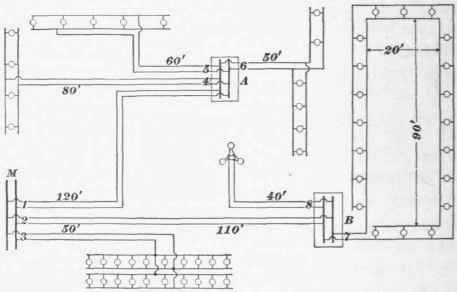A group of different methods of wiring for the two-wire system is shown in Fig. 51. It will be noticed that the closet A is so placed that the lamp feet of the various branch circuits are approximately equal, so that the drop will be uniform in each.Fig. 51.

From the foregoing directions it will be easy to follow out the calculations for the wiring, as embodied in the example below.

## Example

A floor of a building is to be fitted with incandescent lamps as indicated in Fig. 51. The mains enter at M ; a drop of 2.5 volts is allowed between this point and the lamps; on each circuit the length in feet is marked, this being the single distance. The lamps are 110-volt, 16-candlepower. Determine the size of feeders and branches.

## Solution

Feeder No. 1 carries current for the lamps on circuits 4, 5, 6, having a total of 15 lamps. The distance from the main to the closet A is 120 feet, and the lamp feet = 15x120 = 1,800 = N F. The total drop allowed is 2.5 volts, of which we will use here, say, 1.5 volts = E. Then, by formula 5, the resistance per foot of the wire

Rf = 1.5 = .000833 ohm, or .833 ohm per 1,000 feet.

1,800

The nearest size of wire is No. 9. The current passing through the conductor is 15 X.5 = 7.5 amperes, which is much below the underwriters' limit, and is safe.

Subfeeder No : 4 carries current for 4 lamps a distance of 80 feet, and the lamp feet = 320 = N F. The drop in voltage will be the difference between the total drop allowed and that taken for the feeder in series with this subfeeder; then 2.5 - 1.5 = 1 volt = E. The resistance per foot of the wire will be

Rf = 1 = .003125 ohm, or 3.125 ohms per 1,000 feet

This is smaller than No. 14, but we must use No. 14, as it is the smallest allowed (Art. 66). The current is only 4 X .5 = 2 amperes, and No. 14 will carry 10 amperes, and is therefore safe.

Subfeeder No. 5 carries current for 5 lamps a distance of 60 feet, and the lamp feet N F = 300. The drop is the same as for circuit 4, and E = 1. Then the resistance per foot of the wire

Rf = 1 = .00333 ohm, or 3.33 ohms per 1,000 feet.

The nearest wire is again No. 14, and the current is 5 X .5 = 2.5 amperes.

Subfeeder No. 6 supplies current to 6 lamps, and the distance being 50 feet, N F = 300. The drop is 1 volt, and the resistance per foot the same wire, No. 14, being used here also. The current is 6 X .5 = 3 amperes.

Rf = 1 = .00333.

Feeder No. 2 is 110 feet in length, and supplies current to 23 lamps. Then N F = 23 X 110 = 2.530. If we allow a drop of 1.5 volts here, we shall have only 1 volt to allow in circuit 7. But circuit 7 contains actually double the length of wire, and it would be a better plan, therefore, to allow 1.5 volts here, and 1 volt in circuit 2. Then, the wire for circuit 2 has a resistance per foot

Rf = 1 = .000395 ohm, or .395 ohm per 1,000 feet,

2,530 which is between Nos. 5 and 6, either of which will carry the current safely. We regulate our choice by the result of the subsequent calculation on circuit 7.

Subfeeder No. 7 carries current for 20 lamps, arranged in a loop. We have seen (Art. 67) that the distance used in calculation is 90 + 20 = 110 feet = F, then the lamp feet N F= 20x110 = 2,200, and the drop in volts is 1.5 = E. Then the resistance per foot of the conductor

Rf = 1.5 = .000682 ohm,

2,200 or, the resistance per 1,000 feet is .682 ohm, corresponding to a size between Nos. 8 and 9, but nearer No. 8. If we use the larger wire here, No. 8, we should use the smaller of the two in the case of the feeder to this closet, No. 2, which we find should be between Nos. 5 and 6. Therefore, we select No. 6 wire for circuit No. 2. For circuit 7, No. 8 wire is safe, the current being 10 amperes.

Subfeeder No. 8 runs to an electrolier with only 3 lights, the distance being 40 feet. The drop in this line should be 2.5 - 1 = 1.5 volts. The resistance per foot of the wire will then be

Rf = 1.5 = .0125 ohm, or 12.5 ohms per 1,000 feet.

This wire will be much too small to use, and we must take No. 14, although these three lamps will run brighter than the others. We may find the drop from formula 8 : E = .00263 X 120 = .3156 volt, instead of 1.5, so that the lamps will have 1.18 volts excess of pressure. This, however, will not injure them.

Feeder No. 3 carries the current for 20 lamps a distance of 50 feet. The drop is 2.5 volts, and the lamp feet = 1,000 = N F. The resistance per foot of the required conductor

Rf = 2.5 = .0025 ohm, or 2.5 ohms per 1,000 feet.

1,000

No. 14 wire is sufficiently near the size to use, and will carry the current, 10 amperes, safely. Ans.